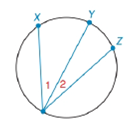Chapter 6.4, Problem 20E### Elementary Geometry for College St...

6th Edition
Daniel C. Alexander + 1 other
ISBN: 9781285195698

#### Solutions

Chapter
Section### Elementary Geometry for College St...

6th Edition
Daniel C. Alexander + 1 other
ISBN: 9781285195698
Textbook Problem
1 views

# a) If m X Y ⌢ > m Y Z ⌢ , write an inequality that compares the measures of inscribed angles 1 and 2 .b) If m ∠ 1 < m ∠ 2 , write and inequality that compares the measures of X Y ⌢ and Y Z ⌢ .To determine

(a)

To write:

An inequality that compares the measures of inscribed angles 1 and 2.

Explanation

Given:

mXY>mYZ and the figure given below

Theorem Used:

According to the following theorem

In a circle (or in congruent circles) containing two unequal arcs, the larger arc corresponds to the larger central angle.

Calculation:

Using following theorem

Since the arcs are unequal i.e. mXY>mYZ and from the given figure

To determine

(b)

To write:

An inequality that compares the measures of XY and YZ.

### Still sussing out bartleby?

Check out a sample textbook solution.

See a sample solution

#### The Solution to Your Study Problems

Bartleby provides explanations to thousands of textbook problems written by our experts, many with advanced degrees!

Get Started

#### Find more solutions based on key concepts